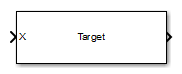•Libraries:
Phased Array System Toolbox / Environment and Target

## Description

The Radar Target block models a radar target that reflects the signal according to the specified radar cross section (RCS). The block supports a non-fluctuating target model and all four Swerling target models. The block does not support polarized signals.

## Ports

### Input

expand all

Incident signal, specified as a complex-valued N-by-1 column vector or complex-valued N-by-M matrix. The value M is the number of signals. Each signal corresponds to a different target. The value N is the number of samples in each signal.

The size of the first dimension of the input matrix can vary to simulate a changing signal length. A size change can occur, for example, in the case of a pulse waveform with variable pulse repetition frequency.

Data Types: `double`

Mean radar cross-section, specified as nonnegative scalar or 1-by-M row vector of nonnegative values. M is the number of targets. This parameter applies to fluctuating and non-fluctuating targets. If you specify M incident signals, you can specify the radar cross-section as a scalar or as a 1-by-M vector. For a scalar, the same value will be applied to all signals. Units are in m².

#### Dependencies

To enable this port, set the Source of mean radar cross section pull down menu to ```Input port```.

Data Types: `double`

Indicator to update radar cross-section for fluctuating targets, specified as 0 or 1. When set to 0, the radar cross-section is constant. When set to 1, the radar cross-section fluctuates.

#### Dependencies

To enable this port, set the Fluctuation model pull down menu to anything except `Nonfluctuating`.

Data Types: `double`

### Output

expand all

Reflected signal, returned as a complex-valued N-by-1 column vector or complex-valued N-by-M matrix. The value M is the number of signals. Each signal corresponds to a different target. The value N is the number of samples in each signal.

## Parameters

expand all

Specify whether the target’s mean radar cross-section (RCS) value comes from the Mean radar cross section parameter of this block or from an input port. Values of this parameter are

 `Property` The Mean radar cross section parameter for this block specifies the mean RCS value. `Input port` Choosing this value creates the `RCS` input port to specify the mean radar cross-section.

Specify the mean value of the target's radar cross section, in square meters, as a nonnegative scalar. This parameter appears only when the Source of mean radar cross section parameter is set to `Property`.

Specify the statistical model of the target as one of `Nonfluctuating`, `Swerling1`, `Swerling2`, `Swerling3`, or `Swerling4`. Setting this parameter to a value other than `Nonfluctuating`, allows setting cross-sections parameters via an input port, `Update`.

Signal propagation speed, specified as a real-valued positive scalar. The default value of the speed of light is the value returned by `physconst('LightSpeed')`.

Data Types: `double`

Specify the carrier frequency of the signal that reflects from the target, as a positive scalar in hertz.

Block simulation, specified as `Interpreted Execution` or `Code Generation`. If you want your block to use the MATLAB® interpreter, choose `Interpreted Execution`. If you want your block to run as compiled code, choose ```Code Generation```. Compiled code requires time to compile but usually runs faster.

Interpreted execution is useful when you are developing and tuning a model. The block runs the underlying System object™ in MATLAB. You can change and execute your model quickly. When you are satisfied with your results, you can then run the block using ```Code Generation```. Long simulations run faster with generated code than in interpreted execution. You can run repeated executions without recompiling, but if you change any block parameters, then the block automatically recompiles before execution.

This table shows how the Simulate using parameter affects the overall simulation behavior.

When the Simulink® model is in `Accelerator` mode, the block mode specified using Simulate using overrides the simulation mode.

Acceleration Modes

 Block Simulation Simulation Behavior `Normal` `Accelerator` `Rapid Accelerator` `Interpreted Execution` The block executes using the MATLAB interpreter. The block executes using the MATLAB interpreter. Creates a standalone executable from the model. `Code Generation` The block is compiled. All blocks in the model are compiled.

 Block Parameter:`SimulateUsing` Type:enum Values:```Interpreted Execution```, `Code Generation` Default:```Interpreted Execution```